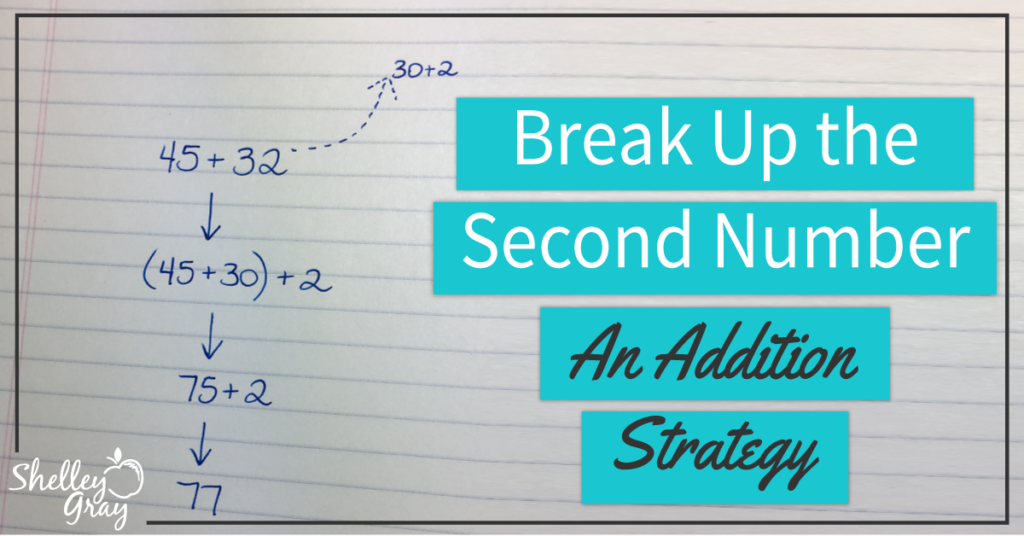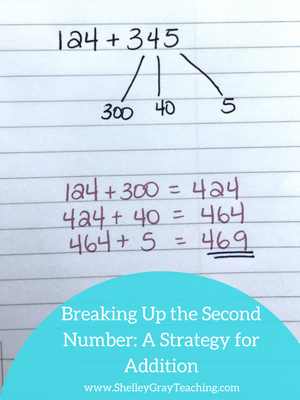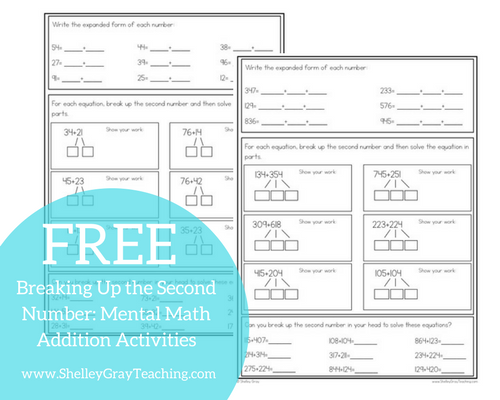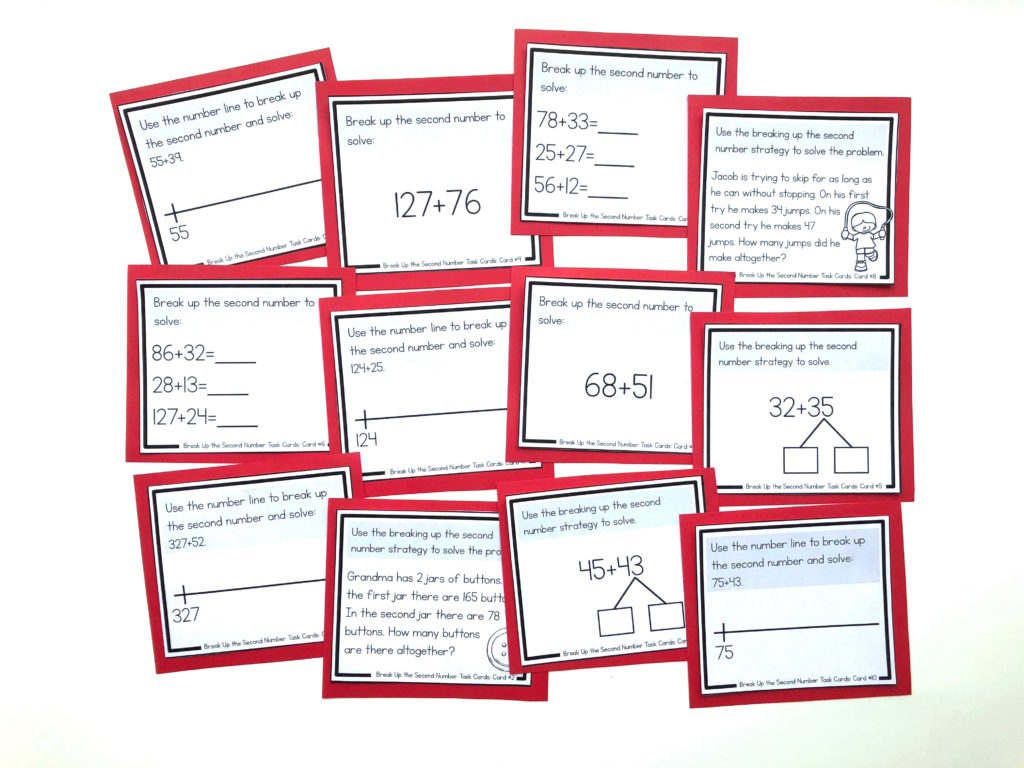# Breaking Up the Second Number: An Addition StrategyBreaking up the second number is a mental math strategy for addition. Some students may find this method more efficient than left-to-right addition.

This strategy involves breaking up the second number in an equation into more manageable parts. Like many other mental math strategies, this strategy encourages students to think flexibly and to manipulate numbers in different ways. This is the big goal of mental math!

As you look at the examples given, you’ll notice that this strategy reinforces place value understanding, as students are breaking the second number into its expanded form.

EXAMPLES

Let’s take a look at how to perform this strategy. Whenever you introduce a new strategy in your classroom, be sure to use small, easy to work with numbers. This will ensure that students can focus on the strategy itself rather than struggling with big numbers while trying to master a new strategy.

In this example, we will add 14+12. We will break the 12 into a 10 and a 2.

Now we add. First we add 14+10 to make 24, and then add the remaining 2 to make 26.Let’s try another example. Here we will solve 35+46. First we break the 46 into a 40 and a 6.

We will add 35+40 to make 75, and then add the remaining 6 to make 81.Breaking up the second number can be used with more digits as well. Let’s try a 3-digit plus 3-digit equation. Here we will add 124+345. We can first break up the 345 into a 300, a 40, and a 5.

Now we begin by adding 124+300 to make 424. Then we will add the 40 to make 464. Lastly we will add the remaining 5 to make 469.FLEXIBLE THINKING

One of the greatest aspects of mental math is that there is not a series of steps to memorize. Really we just want our students to understand what the numbers mean and be able to manipulate them in a way that works for each individual student.

Suppose we have the equation 213+214.

One student might choose to break up the second number and add 213+200+10+4.

Another student might choose to break up the second number into only two parts and add 200+210+4.

A third student might choose to add these numbers using left to right addition.There is no ONE right way. When students know the values of the digits, and understand what the numbers mean, we open up the options for how an equation can be solved.

This is one strategy that you definitely will want to incorporate into your math instruction. It can be used in many different ways and you will notice that your students begin using this sort of thinking for other math concepts as well.

NEXT STEPS:

• If you would like full support for teaching addition strategies in your classroom, check out The Addition Station HEREYou’ll find this strategy in the Addition Stations for the upper grades. These Math Stations are self-paced, student-centered stations for the basic math strategies. Students move through the levels at their own pace, ensuring that they are always challenged, and working to their full potential.